Documentation

### This is machine translation

Mouseover text to see original. Click the button below to return to the English version of the page.

# createSimFunction (model)

Create SimFunction object

## Syntax

``F = createSimFunction(model,params,observables,dosed)``
``F = createSimFunction(model,params,observables,dosed, variants)``
``F = createSimFunction(___,Name,Value)``

## Description

example

````F = createSimFunction(model,params,observables,dosed)` creates a `SimFunction object` `F` that you can execute like a function handle. The `params` and `observables` arguments define the inputs and outputs of the function `F` when it is executed, and `dosed` defines the dosing information of species. See ```SimFunction object``` for details on how to execute `F`.```

example

````F = createSimFunction(model,params,observables,dosed, variants)` creates a `SimFunction object`, applying the values stored in `variants`, a vector of variant objects, as the model baseline values.```

example

````F = createSimFunction(___,Name,Value)` uses additional options specified by one or more `Name,Value` pair arguments.```

### Note

• Active doses and variants of the model are ignored when `F` is executed.

• `F` is immutable after it is created.

• `F` is automatically accelerated at the first function execution. However, manually accelerate the object if you want it accelerated in your deployment applications.

## Input Arguments

collapse all

SimBiology model, specified as a SimBiology `model object`.

Inputs of SimFunction `F`, specified as a character vector, cell array of character vectors, or an empty cell array `{}`. The character vectors represent the names of model quantities (species, compartments, or parameters) that define the inputs of `F`. Use an empty cell array `{}` to create a `SimFunction object` that has no parameters.

To unambiguously name a model quantity, use the qualified name, which includes the name of the compartment. To name a reaction-scoped parameter, use the reaction name to qualify the parameter. If the name is not a valid MATLAB® variable name, surround it by square brackets such as `[reaction 1].[parameter 1]`.

Outputs of SimFunction `F`, specified as a character vector or cell array of character vectors. The character vectors represent the names of model quantities (species, compartments, or parameters) that define the outputs of `F`.

Dosed species or dose objects, specified as a character vector, cell array of character vectors, vector of dose objects, or empty array `[]`. Use `[]` to specify no species are dosed during simulation.

If it is a cell array of character vectors, it must be 1-by-N array, where N is the number of dosed species names. You can use duplicate species names if you plan to use multiple doses for the same species when you run the SimFunction `F`. Using only dosed species names contains no information on the dose properties. If you have a dose object that contains parameterized properties such as `Amount`, use the dose object as input instead of just species names to transfer such parameter information to the created SimFunction `F`.

If it is a vector of dose objects, it must be 1-by-N vector, where N is the number of dose objects. If dose objects have properties with nondefault numeric values, these values are ignored and a warning is issued. Only `TargetName`, `DurationParameterName`, `LagParameterName`, and parameterized properties are used to create the SimFunction object `F`, that is, to define the `Dosed` property of `F`. For details on how the `Dosed` property table is populated, see Property Summary.

The number of dosed species defined here, that is, the `Dosed` property of the created SimFunction object `F`, must be consistent with the dose input argument `u` of the SimFunction `F` when you execute the object. In other words, the number of elements (N) in the `Dosed` property of `F` must be equal to the number of elements (columns) in the cell array `u`. The order of dosed species in `Dosed` must also match the order of dose tables in `u`.

Alternate model values, specified as a variant or vector of variant objects. These values are applied as the model baseline values when the `SimFunction` object is created. If there are multiple variants referring to the same model element, the last occurrence is used.

### Name-Value Pair Arguments

Specify optional comma-separated pairs of `Name,Value` arguments. `Name` is the argument name and `Value` is the corresponding value. `Name` must appear inside quotes. You can specify several name and value pair arguments in any order as `Name1,Value1,...,NameN,ValueN`.

Example: `'UseParallel',true` specifies to execute the SimFunction `F` in parallel.

Flag to execute SimFunction `F` in parallel, specified as the comma-separated pair consisting of `'UseParallel'` and `true` or `false`. If `true` and Parallel Computing Toolbox™ is available, the SimFunction `F` is executed in parallel.

Example: `'UseParallel'`,`true`

Sensitivity output factors, specified as the comma-separated pair consisting of `'SensitivityOutputs'` and a cell array of character vectors. The character vectors are the names of model quantities (species and parameters) for which you want to compute the sensitivities. The default is `{}` meaning there is no output factors. Output factors are the numerators of time-dependent derivatives explained in Sensitivity Calculation.

Use the keyword `'all'` to specify all model quantities listed in the `observables` argument as sensitivity outputs. However, `{'all'}` means a model quantity named `all` in the model.

You must specify both `'SensitivityOutputs'` and `'SensitivityInputs'` name-value pair arguments for sensitivity calculations.

Example: `'SensitivityOutputs'`,`'all'`

Sensitivity input factors, specified as the comma-separated pair consisting of `'SensitivityInputs'` and a cell array of character vectors. The character vectors are the names of model quantities (species, compartments, and parameters) with respect to which you want to compute the sensitivities. The default is `{}` meaning no input factors. Input factors are the denominators of time-dependent derivatives explained in Sensitivity Calculation.

Use the keyword `'all'` to specify all model quantities listed in the `params` argument as sensitivity inputs. However, `{'all'}` means a model quantity named `all` in the model.

You must specify both `'SensitivityOutputs'` and `'SensitivityInputs'` name-value pair arguments for sensitivity calculations.

Example: `'SensitivityInputs'`,`{'Reaction1.c1','Reaction1.c2'}`

Normalization for calculated sensitivities, specified as the comma-separated pair consisting of `'SensitivityNormalization'` and `'None'`, `'Half'`, or `'Full'`.

• `'None'` — No normalization (default)

• `'Half'` — Normalization relative to the numerator only

• `'Full'` — Full dedimensionalization

For details, see `Normalization`.

Example: `'SensitivityNormalization'`,`'Full'`

## Output Arguments

collapse all

SimFunction, returned as a ```SimFunction object``` or ```SimFunctionSensitivity object```. You can execute `F` like a function handle.

`F` is a ```SimFunctionSensitivity object``` if you specify non-empty `'SensitivityOutputs'` and `'SensitivityInputs'` name-value pair arguments.

## Examples

collapse all

This example uses a radioactive decay model with the first-order reaction $\frac{dz}{dt}=c\cdot x$, where `x` and `z` are species and `c` is the forward rate constant.

Load the sample project containing the radioactive decay model `m1`.

`sbioloadproject radiodecay;`

Create a `SimFunction object`, specifying the parameter `Reaction1.c` to be scanned, and species `x` as the output of the function with no dosed species.

`f = createSimFunction(m1, 'Reaction1.c','x', [])`
```f = SimFunction Parameters: Name Value Type Units _____________ _____ ___________ __________ 'Reaction1.c' 0.5 'parameter' '1/second' Observables: Name Type Units ____ _________ __________ 'x' 'species' 'molecule' Dosed: None ```

If the `UnitConversion` option was set to `false` when the `SimFunction` object `f` was created, the table does not display the units of the model quantities.

To illustrate this, first set the `UnitConversion` option to `false`.

```cs = getconfigset(m1); cs.CompileOptions.UnitConversion = false;```

Create the `SimFunction` object as before and note that the variable named `Units` disappears.

`f = createSimFunction(m1, {'Reaction1.c'},{'x'}, [])`
```f = SimFunction Parameters: Name Value Type _____________ _____ ___________ 'Reaction1.c' 0.5 'parameter' Observables: Name Type ____ _________ 'x' 'species' Dosed: None ```

If any of the species in the model is being dosed, specify the names of dosed species as the last argument. For example, if the species `x` is being dosed, specify it as the last argument.

`f = createSimFunction(m1, {'Reaction1.c'},{'x'}, 'x')`
```f = SimFunction Parameters: Name Value Type _____________ _____ ___________ 'Reaction1.c' 0.5 'parameter' Observables: Name Type ____ _________ 'x' 'species' Dosed: TargetName __________ 'x' ```

Once the `SimFunction` object is created, you can execute it like a function handle and perform parameter scans (in parallel if Parallel Computing Toolbox™ is available), Monte Carlo simulations, and scans with multiple or vectorized doses. See `SimFunction object` for more examples.

This example creates a `SimFunction` object with dosing information using a `RepeatDose` or `ScheduleDose` object or a vector of these objects. However, if any dose object contains data such as `StartTime`, `Amount`, and `Rate`, such data are ignored, and a warning is issued. Only data, if available, used are `TargetName`, `LagParameterName`, and `DurationParameterName` of the dose object.

Load the sample project containing the radioactive decay model `m1`.

`sbioloadproject radiodecay;`

Create a `RepeatDose object` and specify its properties.

```rdose = sbiodose('rd'); rdose.TargetName = 'x'; rdose.StartTime = 5; rdose.TimeUnits = 'second'; rdose.Amount = 300; rdose.AmountUnits = 'molecule'; rdose.Rate = 1; rdose.RateUnits = 'molecule/second'; rdose.Interval = 100; rdose.RepeatCount = 2;```

Add a lag parameter and duration parameter to the model.

```lagPara = addparameter(m1,'lp'); lagPara.Value = 1; lagPara.ValueUnits = 'second'; duraPara = addparameter(m1,'dp'); duraPara.Value = 1; duraPara.ValueUnits = 'second';```

Set these parameters to the dose object.

```rdose.LagParameterName = 'lp'; rdose.DurationParameterName = 'dp';```

Create a `SimFunction` object `f` using the `RepeatDose` object `rdose` that you just created.

`f = createSimFunction(m1,{'Reaction1.c'},{'x','z'},rdose)`
```Warning: Some Dose objects in DOSED had data. This data will be ignored. > In SimFunction>SimFunction.SimFunction at 847 In SimFunction>SimFunction.createSimFunction at 374 f = SimFunction Parameters: Name Value Type Units _____________ _____ ___________ __________ 'Reaction1.c' 0.5 'parameter' '1/second' Observables: Name Type Units ____ _________ __________ 'x' 'species' 'molecule' 'z' 'species' 'molecule' Dosed: TargetName TargetDimension __________ _______________________________ 'x' 'Amount(e.g. mole or molecule)' DurationParameterName DurationParameterValue _____________________ ______________________ 'dp' 1 DurationParameterUnits LagParameterName ______________________ ________________ 'second' 'lp' LagParameterValue LagParameterUnits _________________ _________________ 1 'second' ```

A warning message appears because the `rdose` object contains data (`StartTime`, `Amount`, `Rate`) that are ignored by the `createSimFunction` method.

This example shows how to execute different signatures of the `SimFunction object` to simulate and scan parameters of the Lotka-Volterra (predator-prey) model described by Gillespie .

Load the sample project containing the model `m1`.

`sbioloadproject lotka;`

Create a SimFunction object `f` with `c1` and `c2` as input parameters to be scanned, and `y1` and `y2` as the output of the function with no dosed species.

`f = createSimFunction(m1,{'Reaction1.c1', 'Reaction2.c2'},{'y1', 'y2'}, [])`
```f = SimFunction Parameters: Name Value Type ______________ _____ ___________ 'Reaction1.c1' 10 'parameter' 'Reaction2.c2' 0.01 'parameter' Observables: Name Type ____ _________ 'y1' 'species' 'y2' 'species' Dosed: None ```

Define an input matrix that contains values for each parameter (`c1` and `c2`) for each simulation. The number of rows indicates the total number of simulations, and each simulation uses the parameter values specified in each row.

`phi = [10 0.01; 10 0.02];`

Run simulations until the stop time is 5 and plot the simulation results.

`sbioplot(f(phi, 5));`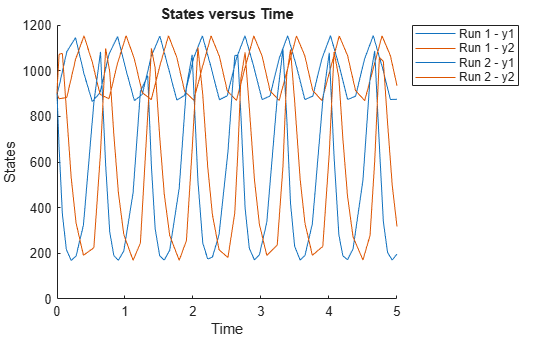You can also specify a vector of different stop times for each simulation.

```t_stop = [3;6]; sbioplot(f(phi, t_stop));```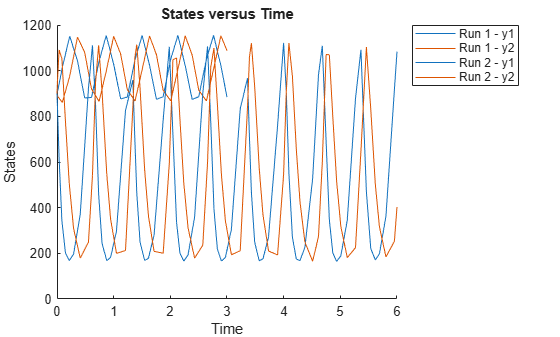Next, specify the output times as a vector.

```t_output = 0:0.1:5; sbioplot(f(phi,[],[],t_output));```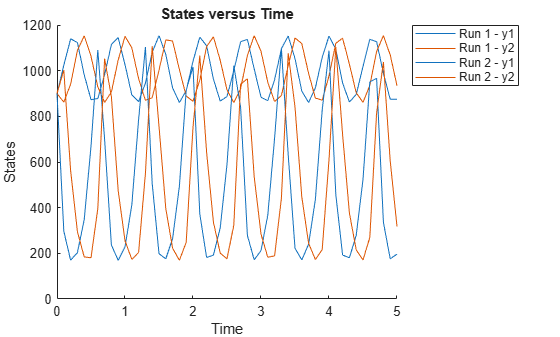Specify output times as a cell array of vectors.

```t_output = {0:0.01:3, 0:0.2:6}; sbioplot(f(phi, [], [], t_output));```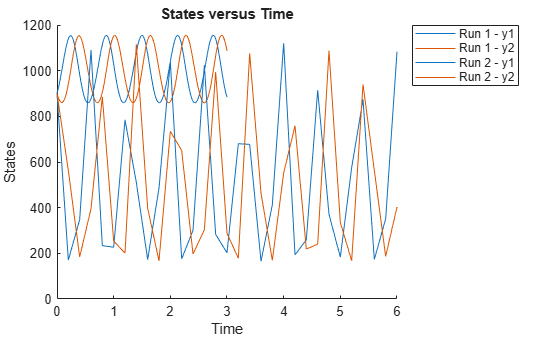This example shows how to calculate sensitivities of some species in the Lotka-Volterra model using the SimFunctionSensitivity object.

`sbioloadproject lotka;`

Define the input parameters.

`params = {'Reaction1.c1', 'Reaction2.c2'};`

Define the observed species, which are the outputs of simulation.

`observables = {'y1', 'y2'};`

Create a `SimFunctionSensitivity` object. Set the sensitivity output factors to all species (`y1` and `y2`) specified in the `observables` argument and input factors to those in the `params` argument (`c1` and `c2`) by using the keyword 'all'.

`f = createSimFunction(m1,params,observables,[],'SensitivityOutputs','all','SensitivityInputs','all','SensitivityNormalization','Full')`
```f = SimFunction Parameters: Name Value Type ______________ _____ ___________ 'Reaction1.c1' 10 'parameter' 'Reaction2.c2' 0.01 'parameter' Observables: Name Type ____ _________ 'y1' 'species' 'y2' 'species' Dosed: None Sensitivity Input Factors: Name Type ______________ ___________ 'Reaction1.c1' 'parameter' 'Reaction2.c2' 'parameter' Sensitivity Output Factors: Name Type ____ _________ 'y1' 'species' 'y2' 'species' Sensitivity Normalization: Full ```

Calculate sensitivities by executing the object with `c1` and `c2` set to 10 and 0.1 respectively. Set the output times from 1 to 10. `t` contains time points, `y` contains simulation data, and sensMatrix is the sensitivity matrix containing sensitivities of `y1` and `y2` with respect to `c1` and `c2`.

`[t,y,sensMatrix] = f([10,0.1],[],[],1:10);`

Retrieve the sensitivity information at simulation time = 5.

```temp = sensMatrix{:}; sensMatrix2 = temp(t{:}==5,:,:); sensMatrix2 = squeeze(sensMatrix2)```
```sensMatrix2 = 2×2 37.6987 -6.8447 -40.2791 5.8225 ```

The rows of `sensMatrix2` represent output factors (`y1` and `y2`). The columns represent the input factors (`c1` and `c2`).

`$sensMatrix2=\left[\begin{array}{cc}\begin{array}{c}\frac{\partial y1}{\partial c1}\\ \\ \frac{\partial y2}{\partial c1}\end{array}& \begin{array}{c}\frac{\partial y1}{\partial c2}\\ \\ \frac{\partial y2}{\partial c2}\end{array}\end{array}\right]$`

Set the stop time to 15, without specifying the output times. In this case, the output times are the solver time points by default.

`sd = f([10,0.1],15);`

Retrieve the calculated sensitivities from the SimData object `sd`.

`[t,y,outputs,inputs] = getsensmatrix(sd);`

Plot the sensitivities of species `y1` and `y2` with respect to `c1`.

```figure; plot(t,y(:,:,1)); legend(outputs); title('Sensitivities of species y1 and y2 with respect to parameter c1'); xlabel('Time'); ylabel('Sensitivity');```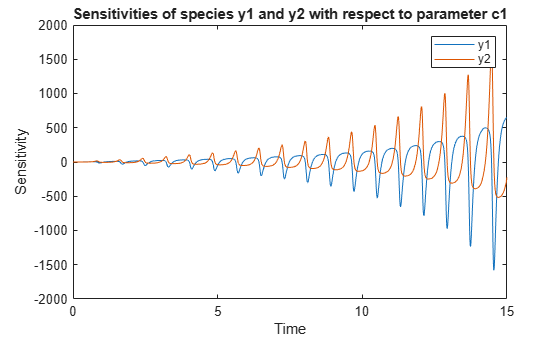Plot the sensitivities of species `y1` and `y2` with respect to `c2`.

```figure; plot(t,y(:,:,2)); legend(outputs); title('Sensitivities of species y1 and y2 with respect to parameter c2'); xlabel('Time'); ylabel('Sensitivity');```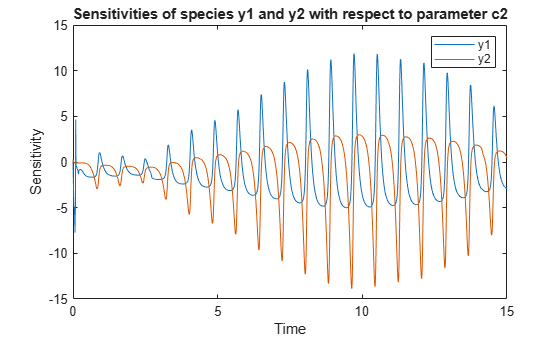Alternatively, you can use `sbioplot`. Expand `Run1` to select which simulation or sensitivity data to display.

`sbioplot(sd);`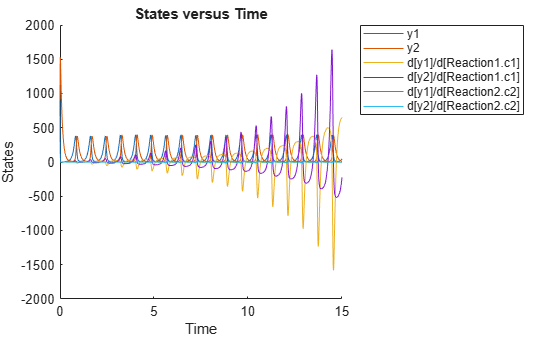You can also plot the sensitivity matrix using the time integral for the calculated sensitivities of `y1` and `y2`. The plot indicates `y1` and `y2` are more sensitive to the parameter `c1` than `c2`.

```[~, in, out] = size(y); result = zeros(in, out); for i = 1:in for j = 1:out result(i,j) = trapz(t(:),abs(y(:,i,j))); end end figure; hbar = bar(result); haxes = hbar(1).Parent; haxes.XTick = 1:length(outputs); haxes.XTickLabel = outputs; legend(inputs,'Location','NorthEastOutside'); ylabel('Sensitivity');```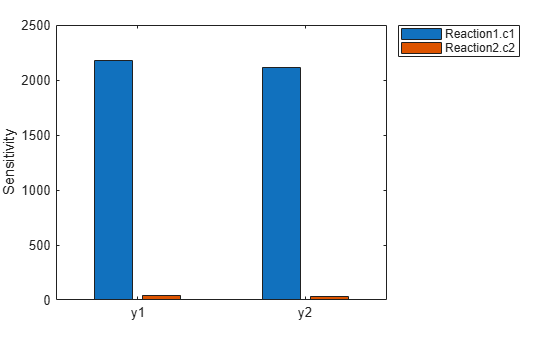This example shows how to simulate the glucose-insulin responses for the normal and diabetic subjects.

Load the model of glucose-insulin response. For details about the model, see the Background section in Simulating the Glucose-Insulin Response.

`sbioloadproject('insulindemo', 'm1')`

The model contains different initial conditions stored in various variants.

`variants = getvariant(m1);`

Get the initial conditions for the type 2 diabetic patient.

`type2 = variants(1)`
``` SimBiology Variant - Type 2 diabetic (inactive) ContentIndex: Type: Name: Property: Value: 1 parameter Plasma Volume ... Value 1.49 2 parameter k1 Value 0.042 3 parameter k2 Value 0.071 4 parameter Plasma Volume ... Value 0.04 5 parameter m1 Value 0.379 6 parameter m2 Value 0.673 7 parameter m4 Value 0.269 8 parameter m5 Value 0.0526 9 parameter m6 Value 0.8118 10 parameter Hepatic Extrac... Value 0.6 11 parameter kmax Value 0.0465 12 parameter kmin Value 0.0076 13 parameter kabs Value 0.023 14 parameter kgri Value 0.0465 15 parameter f Value 0.9 16 parameter a Value 6e-05 17 parameter b Value 0.68 18 parameter c Value 0.00023 19 parameter d Value 0.09 20 parameter Stomach Glu Af... Value 125 21 parameter kp1 Value 3.09 22 parameter kp2 Value 0.0007 23 parameter kp3 Value 0.005 24 parameter kp4 Value 0.0786 25 parameter ki Value 0.0066 26 parameter [Ins Ind Glu U... Value 1 27 parameter Vm0 Value 4.65 28 parameter Vmx Value 0.034 29 parameter Km Value 466.21 30 parameter p2U Value 0.084 31 parameter K Value 0.99 32 parameter alpha Value 0.013 33 parameter beta Value 0.05 34 parameter gamma Value 0.5 35 parameter ke1 Value 0.0007 36 parameter ke2 Value 269 37 parameter Basal Plasma G... Value 164.18 38 parameter Basal Plasma I... Value 54.81 ```

Suppress an informational warning that is issued during simulations.

`warnSettings = warning('off','SimBiology:DimAnalysisNotDone_MatlabFcn_Dimensionless');`

Create SimFunction objects to simulate the glucose-insulin response for the normal and diabetic subjects.

• Specify an empty array `{}` for the second input argument to denote that the model will be simulated using the base parameter values (that is, no parameter scanning will be performed).

• Specify the plasma glucose and insulin concentrations as responses (outputs of the function to be plotted).

• Specify the species `Dose` as the dosed species. This species represents the initial concentration of glucose at the start of the simulation.

```normSim = createSimFunction(m1,{},... {'[Plasma Glu Conc]','[Plasma Ins Conc]'},'Dose')```
```normSim = SimFunction Parameters: Observables: Name Type Units ___________________ _________ _____________________ '[Plasma Glu Conc]' 'species' 'milligram/deciliter' '[Plasma Ins Conc]' 'species' 'picomole/liter' Dosed: TargetName TargetDimension __________ ___________________ 'Dose' 'Mass (e.g., gram)' ```

For the diabetic patient, specify the initial conditions using the variant `type2`.

```diabSim = createSimFunction(m1,{},... {'[Plasma Glu Conc]','[Plasma Ins Conc]'},'Dose',type2)```
```diabSim = SimFunction Parameters: Observables: Name Type Units ___________________ _________ _____________________ '[Plasma Glu Conc]' 'species' 'milligram/deciliter' '[Plasma Ins Conc]' 'species' 'picomole/liter' Dosed: TargetName TargetDimension __________ ___________________ 'Dose' 'Mass (e.g., gram)' ```

Select a dose that represents a single meal of 78 grams of glucose at the start of the simulation.

`singleMeal = sbioselect(m1,'Name','Single Meal');`

Convert the dosing information to the table format.

`mealTable = getTable(singleMeal);`

Simulate the glucose-insulin response for a normal subject for 24 hours.

`sbioplot(normSim([],24,mealTable));`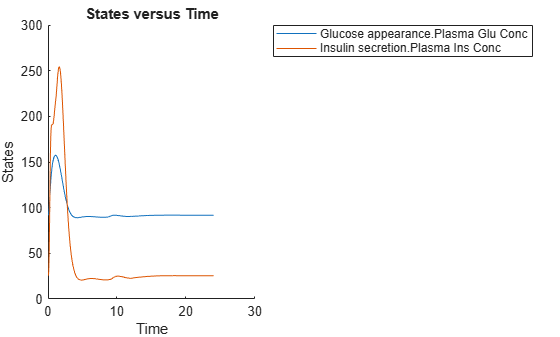Simulate the glucose-insulin response for a diabetic subject for 24 hours.

`sbioplot(diabSim([],24,mealTable));`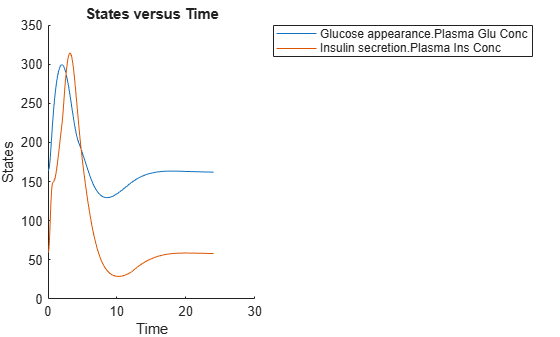Perform a scan using variants

Suppose you want to perform a parameter scan using an array of variants that contain different initial conditions for different insulin impairments. For example, the model `m1` has variants that correspond to the low insulin sensitivity and high insulin sensitivity. You can simulate the model for both conditions via a single call to the SimFunction object.

Select the variants to scan.

```varToScan = sbioselect(m1,'Name',... {'Low insulin sensitivity','High insulin sensitivity'});```

Check which model parameters are being stored in each variant.

`varToScan(1)`
``` SimBiology Variant - Low insulin sensitivity (inactive) ContentIndex: Type: Name: Property: Value: 1 parameter Vmx Value 0.0235 2 parameter kp3 Value 0.0045 ```
`varToScan(2)`
``` SimBiology Variant - High insulin sensitivity (inactive) ContentIndex: Type: Name: Property: Value: 1 parameter Vmx Value 0.094 2 parameter kp3 Value 0.018 ```

Both variants store alternate values for `Vmx` and `kp3` parameters. You need to specify them as input parameters when you create a SimFunction object.

Create a SimFunction object to scan the variants.

```variantScan = createSimFunction(m1,{'Vmx','kp3'},... {'[Plasma Glu Conc]','[Plasma Ins Conc]'},'Dose');```

Simulate the model and plot the results. `Run 1` include simulation results for the low insulin sensitivity and `Run 2` for the high insulin sensitivity.

`sbioplot(variantScan(varToScan,24,mealTable));`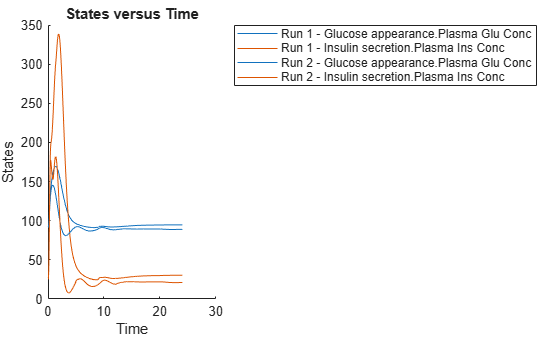Low insulin sensitivity lead to increased and prolonged plasma glucose concentration.

Restore warning settings.

`warning(warnSettings);`

 Gillespie, D.T. (1977). Exact Stochastic Simulation of Coupled Chemical Reactions. The Journal of Physical Chemistry. 81(25), 2340–2361.

##### SupportGet trial now